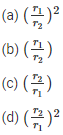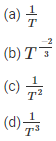Knowledge

# Gravitation MCQs for NEET – NCERT BooksWritten by

NEET Physics is the scoring paper in medical entrance examination. Here, you will get NEET Physics MCQ questions for all concepts as per the latest syllabus. Practice more on a regular basis with these NEET Physics objective questions on Air Pollution and improve your subject knowledge and problem-solving skills along with time management. NEET Physics Gravitation Multiple Choice Questions make you feel confident in answering the question in the exam and increase your score to a higher level.

## mcq on gravity

1. What happens to the gravitational potential at the center of a uniform spherical shell that gradually contracts?
(A) remains constant
(B) decreases
(c) increases
(d) oscillates

2. If the satellite is near the Earth’s surface, what is the relationship between the escape velocity and the orbital velocity of the satellite?Answer: (B) $$v_{e}=\sqrt{2} v_Education$$

3. If the weight of the body at a certain time becomes $$\frac{1}{16}$$, then what will be the effect on its weight. Also, consider the radius of the Earth, R.
(A)4R
(B) 15R
(c) 5r
(d) 3r

4. If the mass of the rocket is M, and the initial speed is V, then what is the maximum height attained by the rocket above the earth’s surface. Let R be the radius of the Earth.Answer: (B) $$\frac{R}{\frac{2 g R}{v^{2}}-1}$$

5. What will be the increase in the potential energy of a body of mass m if the body is taken to a height h which is equal to the radius of the Earth?
(A) MGR
(B) 2MGR
(c) $$\frac{1}{2}$$mgR
(d) $$\frac{1}{4}$$mgR

Answer: (c) $$\frac{1}{2}$$mgR

6. Consider a body of mass m which needs to be transferred from an orbit of radius 2R to 3R. how much energy is requiredAnswer: (B) $$\frac{GM m}{6 R}$$

7. The distance between the nearest planet and the Sun is r1 while the distance between the farthest planet and the Sun is r2. The linear velocities of these planets are v1 and v2 respectively. What is the ratio of $$\frac{v_{1}}{v_{2}}$$Answer: (C) $$\left(\frac{r_{2}}{r_{1}}\right)$$

8. Select the factor on which the orbital velocity does not depend when the satellite is orbiting close to the Earth’s surface
(a) mass of the Earth
(b) mass of the satellite

9. If a satellite takes T time to rotate, the kinetic energy is proportional toAnswer: (B) $$T^{\frac{-2}{3}}$$

10. What holds the atmosphere around the Earth?
(a) gravity
(b) winds
(c) clouds
(d) none of the above